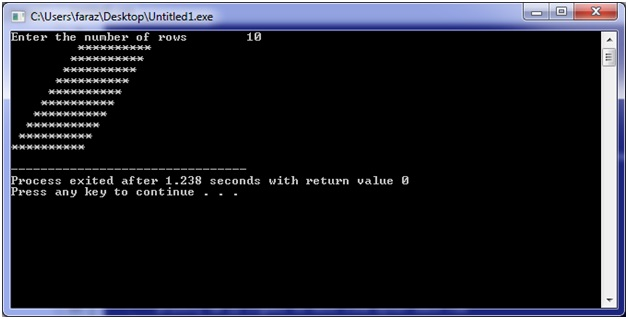# C program to print 'Rhombus' pattern

In this C program, we are implementing logic to print Rhombus pattern using asterisks and loops (nested loops).
Submitted by Shamikh Faraz, on February 16, 2018

Consider the program, to print ‘Rhombus’ pattern

C program

﻿

```#include <stdio.h>

int main() {
int a,b,c, rows;

printf("Enter the number of rows\t");
scanf("%d", &rows);

for(a=1; a<=rows; a++)
{
// Print margin spaces before the stars in each row
for(b=a; b<rows; b++)
{
printf(" ");
}

// Print spaces after the stars in each row
for(c=1; c<=rows; c++)
{
printf("*");
}
//goes to next line after each row
printf("\n");
}
return 0;
}
```

Output